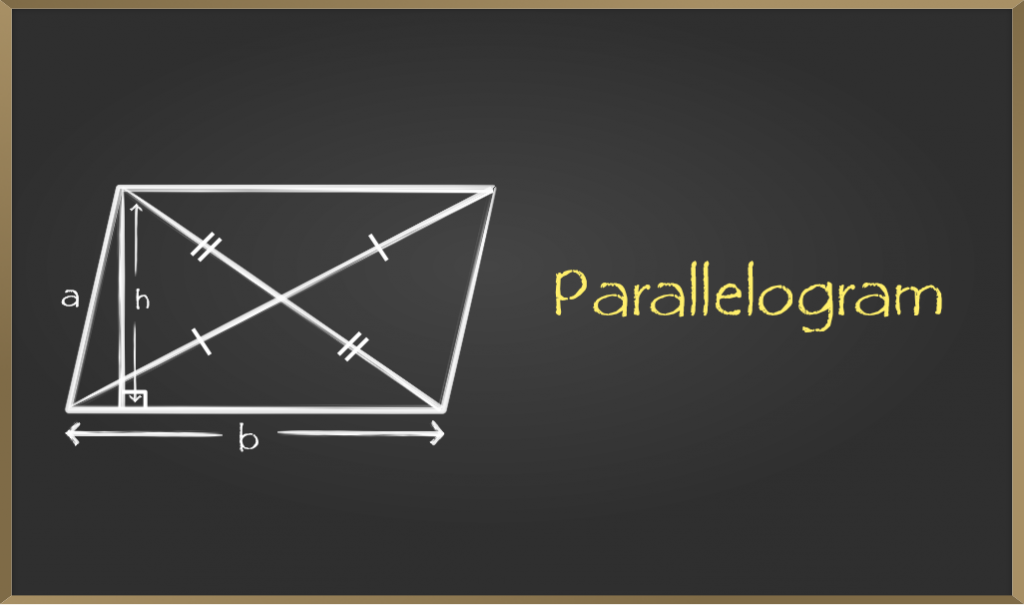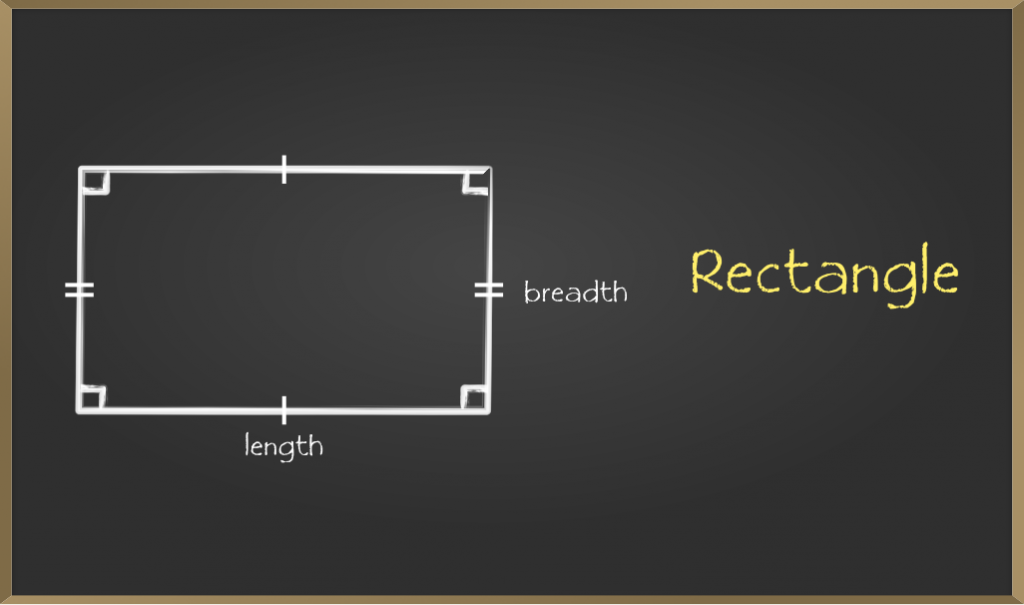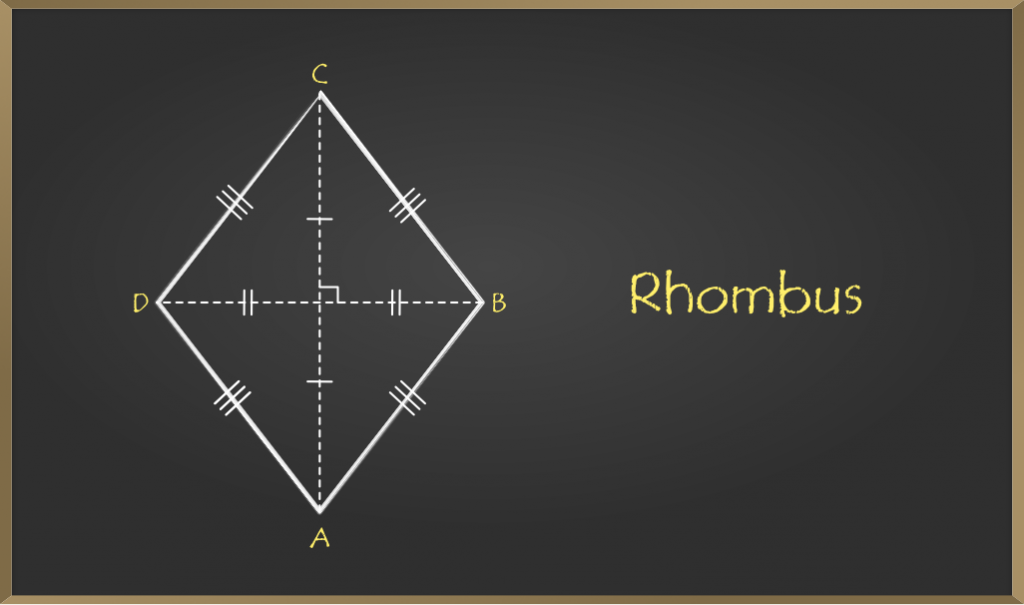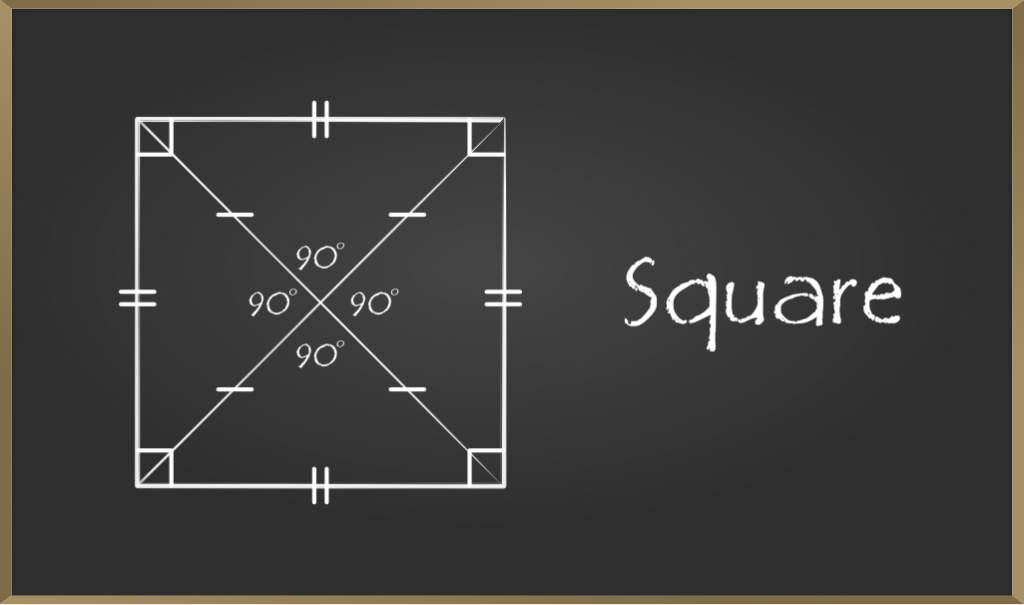# Types of Quadrilaterals – Some Special Parallelograms

• Last Updated : 21 Dec, 2020

Quadrilaterals can be defined as, types of polygons that have four sides, four vertices, and four angles along with a pair of diagonals. The Sum of interior angles of quadrilaterals is 360°. There are various kinds of quadrilaterals. As the name itself suggest the word is a combination of two Latin words ‘Quadri‘ means a variant of four, and ‘latus‘ means side. In this topic, we are going to study some special types of parallelogram like rectangle, square, rhombus.

A parallelogram is a special type of quadrilateral whose opposite side are equal and parallel.

Attention reader! All those who say programming isn't for kids, just haven't met the right mentors yet. Join the  Demo Class for First Step to Coding Coursespecifically designed for students of class 8 to 12.

The students will get to learn more about the world of programming in these free classes which will definitely help them in making a wise career choice in the future.### Properties of Parallelogram

There are different properties of parallelograms.

1. Opposite sides are equal.
2. Opposite angle are equal
3. Diagonals bisect each other.
4. A diagonal of a parallelogram divides it into two congruent triangles.

### Some Special Parallelograms

Depending on the properties, there are three special types of parallelogram:

• Rectangle
• Rhombus
• Square

## Rectangle

A rectangle is a special type of parallelogram which has all the properties of parallelogram along with some different properties. Each angle of a rectangle must be a right angle, i.e. of 90°.### Properties of a Rectangle

Properties of a rectangle are similar to those of a parallelogram:

• Opposite Sides are parallel to each other.
• Opposite Sides of a rectangle are equal.
• Diagonals bisect each other
• Diagonals of the rectangle are equal.
• Each interior angle of a rectangle is equal i.e. 90°

Note: Rectangle has all the properties of parallelogram. So all rectangles are parallelogram but all parallelograms are not rectangle.

### Sample Problems on Rectangles

Problem 1: In rectangle ABCD, AO = 5cm. Find the length of diagonal BD. Also find the perimeter of rectangle if AB = 8cm and AD = 6cm.

Solution:

AO = OC = 5cm (diagonals bisect each other)

Therefore, AC = 10cm

BD = AC =10cm (diagonals of rectangle are equal)

Perimeter = AB + BC + CD + DA

=8 + 6 +8 +6 (opposite sides are equal)

= 28cm

Problem 2: In rectangle ABCD, ∠ABD = 3x – 7 and ∠CBA = 6x – 2. Find the value of x.

Solution:

Each angle of rectangle is 900

Therefore,

∠ABD + ∠CBA = 900

3x – 7 + 6x – 2 = 90

9x – 9 = 90

9x = 99

x = 11

Problem 3: In rectangle ABCD AO = 2x – 10 cm, OB = x + 4 cm. Find the length of diagonal BD

Solution:

In rectangle diagonals bisect each other and are equal.

Therefore, AO = OB

2x – 10 = x + 4

x = 14

OB = 14 + 4 = 18 cm

OD = 18 cm (as diagonals bisect each other)

Therefore, BD = 36 cm

## Rhombus

A quadrilateral that has all sides equal and opposite sides parallel is called Rhombus. Opposite angles of a rhombus are equal and diagonals of the Rhombus bisect each other perpendicularly. Note all rhombus are parallelograms but the reverse of this is not true.### Properties of a Rhombus

Properties of a rhombus are similar to those of a parallelogram:

• Opposite Sides are parallel to each other.
• All the sides of a rhombus are equal to each other.
• Diagonals bisect each other
• Opposite angles of a rhombus are equal.

Note: Rhombus is a parallelogram with all side equal

### Sample Problems on Rhombus

Problem 1: Diagonals of rhombus are 24cm and 10cm. Find the side of rhombus.

Solution:

AC = 24cm

BD = 10cm

Therefore, AO = 12cm and OB = 5cm (diagonals bisect each other)

In right-angled triangle AOB, (diagonals of rhombus are perpendicular)

AB2 = OA2 + OB2

AB2 = 122 + 52

AB2 = 144 + 25

AB2 = 169

AB = 13cm

Therefore, side of rhombus is 13cm.

Problem 2: In a rhombus one of the diagonals is equal to a side of the rhombus. Find the angles of rhombus.

Solution:

In rhombus PQRS PR = PQ (given)

Therefore, PQ = QR = RS = SP = PR (as all side of rhombus are equal)

In triangle PQR

PQ = QR = PR

Therefore, it is an equilateral triangle.

∠QPR = ∠Q = ∠QRP = 600

||ly ∠SPR = ∠S = ∠PRS = 600

Therefore, angles of rhombus are ∠P = 1200 , ∠Q = 600, ∠R = 1200, ∠S = 600

Problem 3: Derive the formula for are of rhombus.

Solution:

As diagonals of rhombus bisect each other at right angle.

In rhombus ABCD

area of triangle ABD = 1/2 * BD *AO (1/2 * base *height) ……….  (1)

area of triangle BCD = 1/2 * BD * CO ………………………………………….. (2)

Area of rhombus = Area of triangle ABD + area of triangle BCD

= 1/2 * BD * AO + 1/2 * BD * CO

= 1/2 * BD (AO + CO)

= 1/2 * BD * AC           (AE + CE = AC)

Therefore, area of rhombus = 1/2 * product of diagonals

## Square

A quadrilateral that has all sides equal and opposite sides parallel and all interior angles equal to 90° is called Square. Diagonals of a square bisect each other perpendicularly. Note that all squares are rhombus but not vice-versa### Properties of a Square

Properties of a square are similar to those of a rhombus:

• Opposite Sides are parallel to each other.
• All the sides of a square are equal to each other.
• Diagonals are perpendicular bisector of each other and are equal.
• All the angles of a square are equal and of 90° each.

### Sample Problems on Square

Problem 1: All rhombus are squares or all squares are rhombus. Which of these statements is correct and why?

Solution:

Square and rhombus both have all sides equal but a rhombus is called square if each of its angle is 900. So all squares can be called rhombus but converse is not true.

Problem 2: In the figure ROPE is a square. Show that diagonals are equal.

Solution

In Δ REP and Δ OEP

RE = OP (sides of square)

∠E = ∠P (each 900)

EP = EP (common)

Therefore, triangles are congruent by SAS criteria.

Therefore, RP = OE (c.p.c.t)

Therefore, diagonals of square are equal.

My Personal Notes arrow_drop_up